# 数据的类型：离散和连续变量

int <- floor(runif(1000,1,7))
ch <- data.frame(char=letters[int],int)
ggplot(ch,aes(int, fill=char)) + geom_bar()
## stat_bin: binwidth defaulted to range/30. Use 'binwidth = x' to adjust this.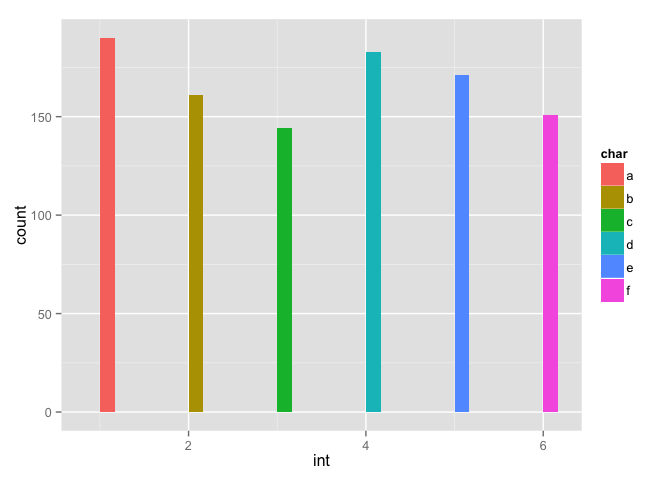stat_bin: binwidth defaulted to range/30. Use ‘binwidth = x’ to adjust this.

ggplot(ch,aes(factor(int), fill=char)) + geom_bar()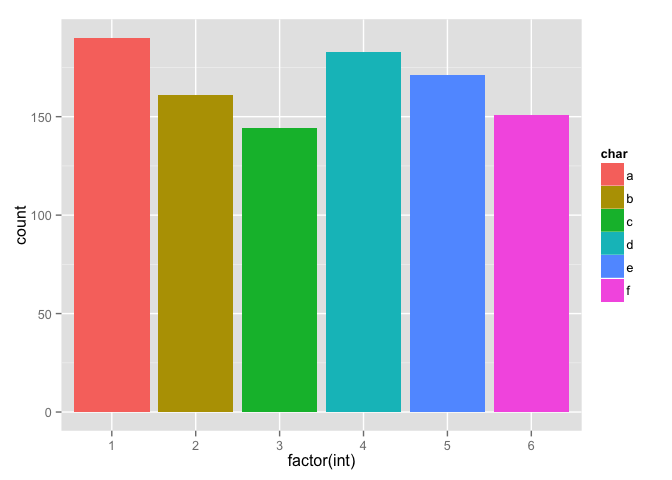ggplot(ch,aes(char, fill=int)) + geom_bar()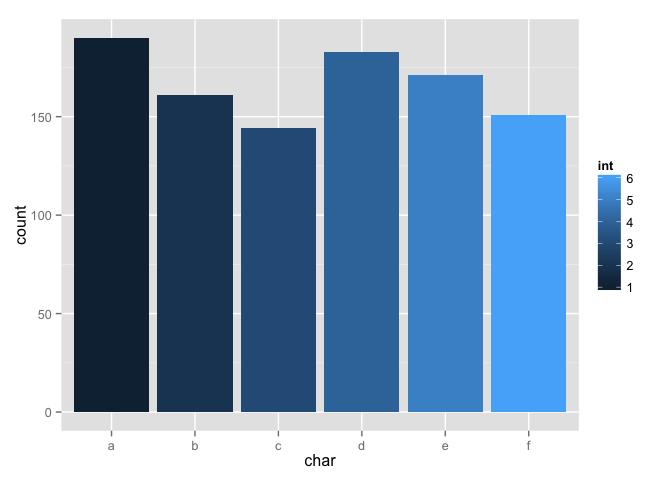ggplot(ch,aes(char, fill=factor(int))) + geom_bar()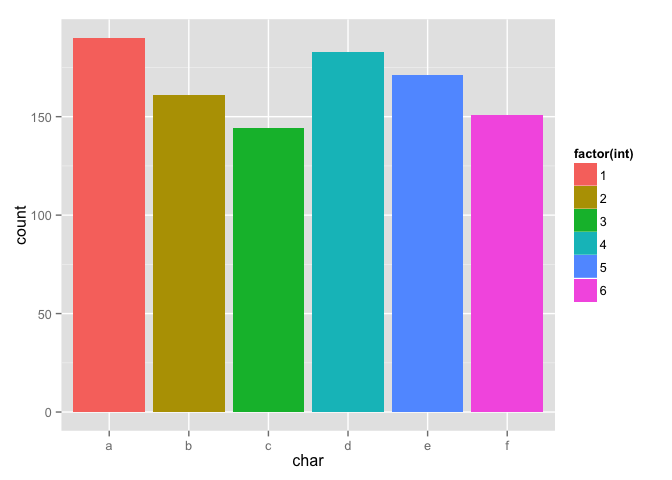# 层：灵活性的源泉

【提示】：以下内容不是官方解释，是笔者的自我感觉，可能不甚准确，欢迎批评指正。

p <- ggplot(mtcars,aes(wt, mpg, size=gear, color=cyl))
p + geom_point() + geom_smooth(method='lm', size=1)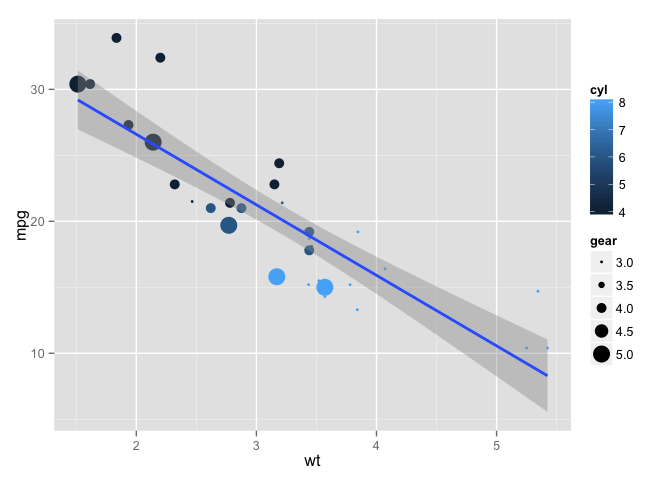p <- ggplot(mtcars,aes(wt, mpg, size=gear, color=factor(cyl)))
p + geom_point() + geom_smooth(method='lm', size=1)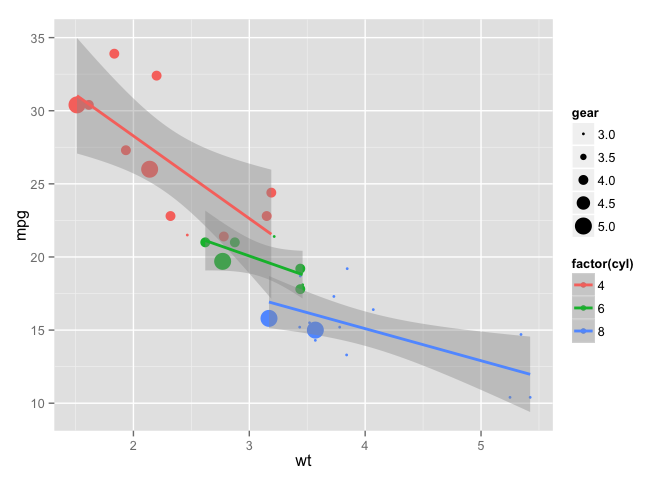p <- ggplot(mtcars,aes(wt, mpg, size=factor(gear)
, color=factor(cyl)))
p + geom_point() + geom_smooth(method='lm', size=1)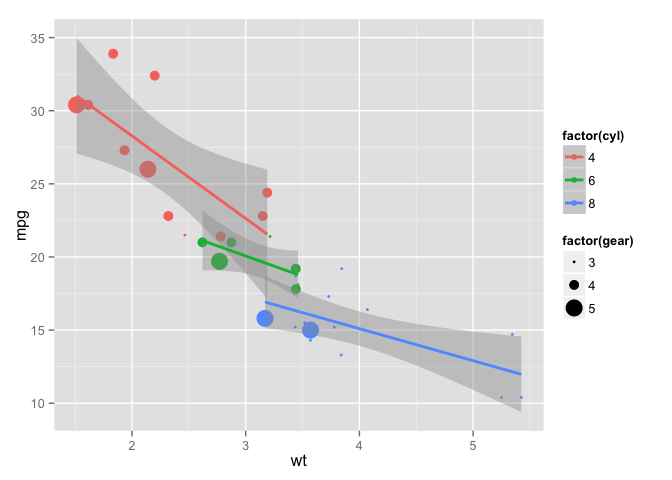p <- ggplot(mtcars,aes(wt, mpg, size=factor(gear)
, color=factor(cyl)))
p + geom_point() + geom_smooth(method='lm')
## Warning in qt((1 - level)/2, df): NaNs produced
## Warning in qt((1 - level)/2, df): NaNs produced
## Warning in qt((1 - level)/2, df): NaNs produced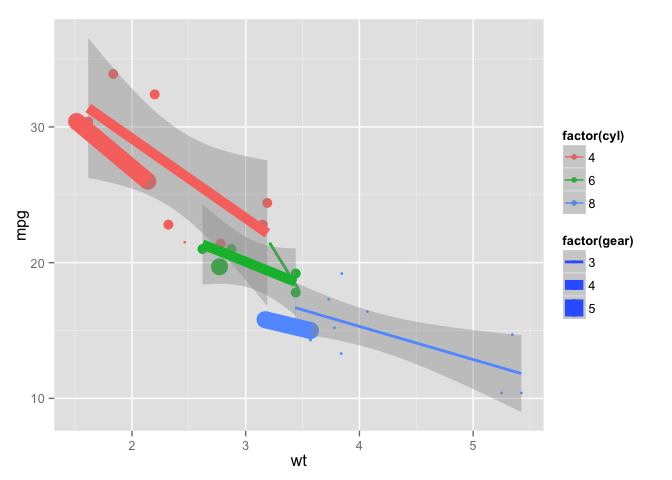p <- ggplot(mtcars,aes(wt, mpg, size=factor(gear)
, color=factor(cyl)))
p <- p + geom_point() + geom_smooth(method='lm')

print(str(p))
## List of 9
##  $data :'data.frame': 32 obs. of 11 variables: ## ..$ mpg : num [1:32] 21 21 22.8 21.4 18.7 18.1 14.3 24.4 22.8 19.2 ...
##   ..$cyl : num [1:32] 6 6 4 6 8 6 8 4 4 6 ... ## ..$ disp: num [1:32] 160 160 108 258 360 ...
##   ..$hp : num [1:32] 110 110 93 110 175 105 245 62 95 123 ... ## ..$ drat: num [1:32] 3.9 3.9 3.85 3.08 3.15 2.76 3.21 3.69 3.92 3.92 ...
##   ..$wt : num [1:32] 2.62 2.88 2.32 3.21 3.44 ... ## ..$ qsec: num [1:32] 16.5 17 18.6 19.4 17 ...
##   ..$vs : num [1:32] 0 0 1 1 0 1 0 1 1 1 ... ## ..$ am  : num [1:32] 1 1 1 0 0 0 0 0 0 0 ...
##   ..$gear: num [1:32] 4 4 4 3 3 3 3 4 4 4 ... ## ..$ carb: num [1:32] 4 4 1 1 2 1 4 2 2 4 ...
##  $layers :List of 2 ## ..$ :Classes 'proto', 'environment' <environment: 0x7f9fad1e1840>
##   ..$:Classes 'proto', 'environment' <environment: 0x7f9fad239808> ##$ scales     :Reference class 'Scales' [package "ggplot2"] with 1 fields
##   ..$scales: list() ## ..and 21 methods, of which 9 are possibly relevant: ## .. add, clone, find, get_scales, has_scale, initialize, input, n, ## .. non_position_scales ##$ mapping    :List of 4
##   ..$x : symbol wt ## ..$ y     : symbol mpg
##   ..$size : language factor(gear) ## ..$ colour: language factor(cyl)
##  $theme : list() ##$ coordinates:List of 1
##   ..$limits:List of 2 ## .. ..$ x: NULL
##   .. ..$y: NULL ## ..- attr(*, "class")= chr [1:2] "cartesian" "coord" ##$ facet      :List of 1
##   ..$shrink: logi TRUE ## ..- attr(*, "class")= chr [1:2] "null" "facet" ##$ plot_env   :<environment: R_GlobalEnv>
##  $labels :List of 4 ## ..$ x     : chr "wt"
##   ..$y : chr "mpg" ## ..$ size  : chr "factor(gear)"
##   ..\$ colour: chr "factor(cyl)"
##  - attr(*, "class")= chr [1:2] "gg" "ggplot"
## NULL

## 展开本分类索引